# Trio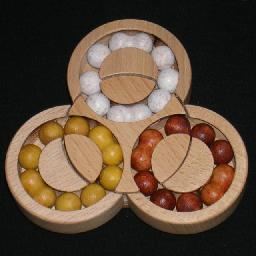This wooden puzzle by Clever Toys has three separate circular tracks arranged in a triangle. The tracks contain ten coloured balls each. The balls can slide along the tracks so each ring can rotate. In the centre of the puzzle is a disc, that can rotate. Each track goes partially through the disc, so when the disc is rotated four balls of each ring get moved to the next ring. Solving the puzzle is made more difficult by the fact that in each ring two pairs of balls are joined together. If such a double piece lies across the seam that separates the middle disc from the rest, the disc cannot move.

The balls come in three colours. In its solved state every ring is a single colour. Furthermore, the bandaged pieces in a ring should be on opposing sides, with three single balls between them on either side.

This puzzle should not be confused with another puzzle of the same name, which you can see on the Turnstile / Puzzler page.

## The number of positions:

Let's count the number of arrangements the bandaged pairs can have. The six pieces can be arranged amongst the rings in the several ways, and the table below tallies them systematically.

Distribution amongst rings Arrangements per ring Total
0+1+5, 0+5+1, 1+0+5, 1+5+0, 5+0+1, 5+1+0 1, 10, 2 6·1·10·2 = 120
0+2+4, 0+4+2, 2+0+4, 2+4+0, 4+0+2, 4+2+0 1, 35, 25 6·1·35·25 = 5,250
0+3+3, 3+0+3, 3+3+0 1, 50, 50 3·1·50·50 = 7,500
1+1+4, 1+4+1, 4+1+1 10, 10, 25 3·10·10·25 = 7,500
1+2+3, 1+3+2, 2+1+3, 2+3+1, 3+1+2, 3+2+1 10, 35, 50 6·10·35·50 = 105,000
2+2+2 35, 35, 35 1·35·35·35 = 42,875
168,245

Given any one of these 168,245 arrangements, we now have to count in how many ways we can assign the colours to the pieces. The 6 bandaged pieces have 2 of each colour, so can have 6!/2!3 colour patterns. The 18 single balls have 6 of each colour, and hence 18!/6!3 colour patterns. Combining all these numbers we get a grand total of 168245·6!/2!3·18!/6!3 = 259,733,642,968,800 positions. Note that this is a slight overestimate. Some positions have one or more rings with a repeating arrangement and colour pattern. The solved position for example remains unchanged if any ring is given a half turn, so it is counted 2·2·2 times in our total. The vast majority of positions do not have such symmetry, so the number is pretty accurate.

## Notation:

A clockwise shift of the upper, left, or right ring by one ball will be denoted by the letters U, L, and R respectively. Anti-clockwise shifts have the letter followed by an apostrophe, i.e. they are denoted by U', L', and R'. Similarly rotations of the middle disc are denoted by M and M'.

## Solution:

Phase 1: Arrange the double pieces.

1. Arrange the double pieces so that there are two in each ring. It doesn't matter which colour they are, or where they are in each ring.
2. Rotate the rings so that no double pieces block the rotation of the centre disc.
3.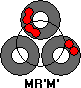If there are is a ring with two adjacent double pieces, then:
1. Hold the puzzle with that ring at the top.
2. Turn the top ring to bring the two doubles to the left, one on either side of the seam.
3. Turn the right ring so that there are at least two single balls at the top to the right of the seam.
4. Do M R' M'.
4. Repeat step c until all doubles are separated by one or more balls.
5.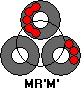If there are is a ring that has two double pieces with a single ball between them, then:
1. Hold the puzzle with that ring at the top.
2. Turn the top ring to bring the sandwiched single ball to the bottom left of the ring, adjacent the seam.
3. Turn the right ring so that there are at least three single balls at the top to the right of the seam.
4. Do M R' M'.
6. Repeat step e until all doubles are separated by two or more balls.
7.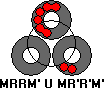If there are is a ring that has two double pieces with exactly two single balls between them, then:
1. Hold the puzzle with that ring at the top.
2. Turn the top ring so that one of the doubles lies at the top of the ring and the other at the bottom-left adjacent to the seam.
3. Turn the right ring so that there are at least two single balls at the bottom to the right of the seam.
4. Do MRRM' U MR'R'M'
8. Repeat step g until all doubles are separated by three balls.
9. Rotate the rings so that each has one double piece at the centre of the puzzle and one at the outer edge.

Phase 2: Solve the double pieces.
In this phase use only half turns of the rings so that the arrangement of the pieces will remain the same.

1. Put a white double at the top of the top ring.
2. Put a yellow double at the outside of the left ring.
3. Put a brown double at the outside of the right ring.
4. Turn the centre so that its white double lies in the top ring.
5. If the other two centre doubles need to be swapped, then do R5 M' R5 M, where R5 is a shift of five balls, i.e. a 180 degree turn.

Phase 3: Solve the single balls.

1.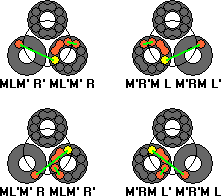Find two unsolved balls that you wish to swap, at least one of which should be next to a double piece.
2. Hold the puzzle so that those two balls lie in the left and right rings.
3. If the chosen ball that is next to a double does not lie in the centre disc, then give that ring a half turn.
4. Rotate the ring with the other ball so that it lies adjacent to the centre disc, and such that a turn of the disc would make the two incorrect balls adjacent.
5. The situation should match one of the pictures on the right. Depending on where the chosen ball on the centre disc is, do one of the following:
1. Left side of the right ring: MLM' R' ML'M' R
2. Top side of the right ring: ML'M' R MLM' R'
3. Right side of the left ring: MR'M' L MRM' L'
4. Top side of the left ring: MRM' L' MR'M' L
6. Repeat steps a-e as often as possible.
7.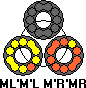The only case left is when none of the incorrect balls are adjacent to a double piece. Choose two of the incorrect balls that you want to swap.
8. Hold the puzzle so that those two balls lie in the left and right rings.
9. Rotate the left ring so that its incorrect ball lies at the bottom right, just below the seam.
10. Rotate the right ring so that its incorrect ball lies at the top right, just to the right of the seam.
11. Do ML'M'L M'R'MR.
12. Go back to step a for any remaining unsolved balls.